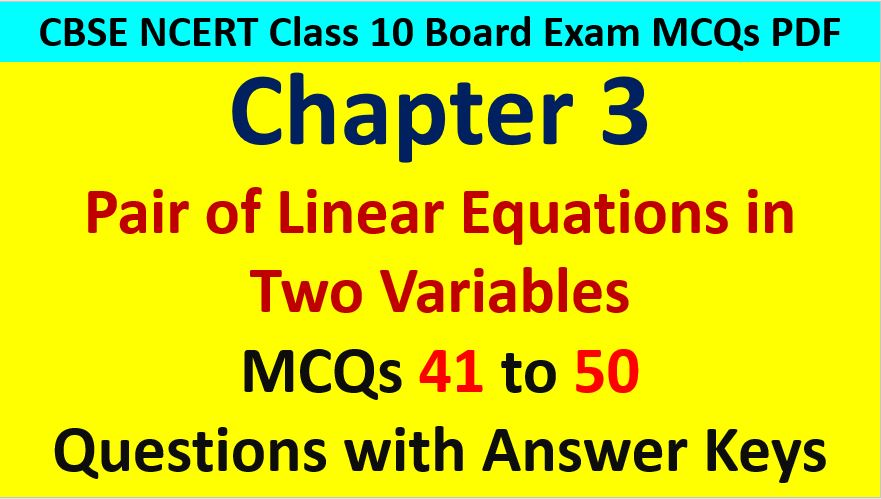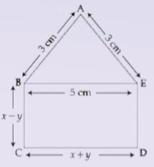Friday, October 22, 2021
Home > CBSE Class 10 > CBSE Class 10 Math Linear Equations in Two Variables MCQ Questions with Answer Keys

# CBSE Class 10 Math Linear Equations in Two Variables MCQ Questions with Answer KeysHi students, Welcome to AMBiPi (Amans Maths Blogs). In this article, you will get CBSE Class 10 Math Linear Equations in Two Variables MCQ Questions with Answer Keys. You can download this PDF and save it in your mobile device or laptop etc.

Linear Equations in Two Variables CBSE Class 10 MCQ Question No 41:

In the figure below, ABCDE is a pentagon with BE || CD and BC || DE. BC is perpendicular to CD. If the perimeter of ABCDE is 21 cm, find the values of x and y (in cm) respectively.Option A : 0, 5

Option B : 5, 0

Option C : 3, 2

Option D : 2, 5

Option B : 5, 0

Linear Equations in Two Variables CBSE Class 10 MCQ Question No 42:

The income of X and Y are in the ratio of 8 : 7 and their expenditure are in the ratio 19 : 16. If each saves Rs. 1250, find their incomes.

Option A : Rs. 6040, Rs. 5285

Option B : Rs. 6000, Rs. 5250

Option C : Rs. 5920, Rs. 5180

Option D : Rs. 5880, Rs. 5185

Option B : Rs. 6000, Rs. 5250

Linear Equations in Two Variables CBSE Class 10 MCQ Question No 43:

A person invested a certain amount at the rate of 12% simple interest and some other amount at the rate of 10% simple interest. He received yearly interest of Rs. 130. But, if he had interchanged the amount invested, he would have received Rs. 4 more as interest. How much did he invest at different rates?

Option A : Rs. 500 at 12%, Rs. 700 at 10%

Option B : Rs. 500 at 12%, Rs. 750 at 10%

Option C : Rs. 550 at 12%, Rs. 700 at 10%

Option D : None of these

Option A : Rs. 500 at 12%, Rs. 700 at 10%

Linear Equations in Two Variables CBSE Class 10 MCQ Question No 44:

The system of equations : x + 2y = 6 and 3x + 6y = 18

Option A : is inconsistent

Option B : has a unique solution

Option C : has infinite number of solutions

Option D : None of these

Option D : has infinite number of solutions

Linear Equations in Two Variables CBSE Class 10 MCQ Question No 45:

4 men and 6 boys can finish a piece of work in 5 days while 3 men and 4 boys can finish it in 7 days. Find the time taken by 1 man alone or that 1 boy alone.

Option A : 30 days, 35 days

Option B : 35 days, 70 days

Option C : 40 days, 65 days

Option D : 65 days, 70 days

Option B : 35 days, 70 days

Linear Equations in Two Variables CBSE Class 10 MCQ Question No 46:

Points A and B are 90 km apart from each other on a highway. A car starts from A and another from B at the same time. If they go in the same direction they meet in 9 hours and if they go in opposite direction they meet in 9/7 hours, Speed of the car starting from point A is

Option A : 30 km/hr

Option B : 90 km/hr

Option C : 40 km/hr

Option D : 220 km/hr

Option C : 40 km/hr

Linear Equations in Two Variables CBSE Class 10 MCQ Question No 47:

From a stationary shop, Sudhir bought two books of Mathematics and three books of Physics of class X for Rs. 850 and Suman bought three books of Mathematics and two books of Physics of Class X for Rs. 900. Find the price of one Mathematics and one Physics book respectively.

Option A : Rs. 150, Rs. 200

Option B : Rs. 145, Rs. 225

Option C : Rs. 225, Rs. 145

Option D : Rs. 200, Rs. 150

Option C : Rs. 200, Rs. 150

Linear Equations in Two Variables CBSE Class 10 MCQ Question No 48:

A two digit number is obtained by either multiplying the sum of the digits by 8 and adding 1 or by multiplying the difference of the digits by 13 and adding 2. Find the number. How many such numbers are there?

Option A : Only One, 41

Option B : Only Two, 41 and 42

Option C : Only One, 43

Option D : None of these

Option A : Only One, 41

Linear Equations in Two Variables CBSE Class 10 MCQ Question No 49:

The unit digit of a two digit number is twice its ten’s digit. If the digits are reversed, the number is 27 more than the original number. Find the number.

Option A : 36

Option B : 40

Option C : 38

Option D : 32

Option A : 36

Linear Equations in Two Variables CBSE Class 10 MCQ Question No 50:

A boatman rows his boat 35 km upstream and 55 km downstream in 12 hours. He can row 30 km upstream and 44 km downstream in 10 hours. Find the total time taken by the boatman to row 50 km upstream and 77 downstream.

Option A : 16 hour

Option B : 17 hour

Option C : 18 hour

Option D : None of these